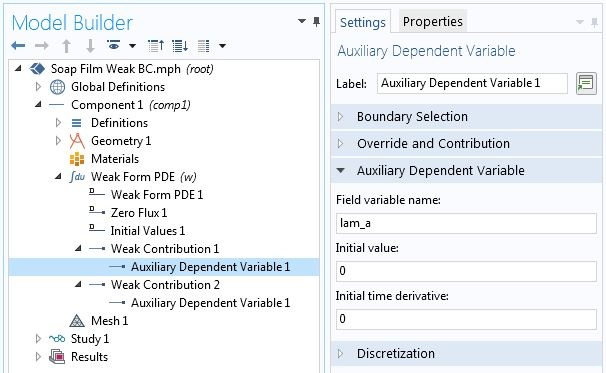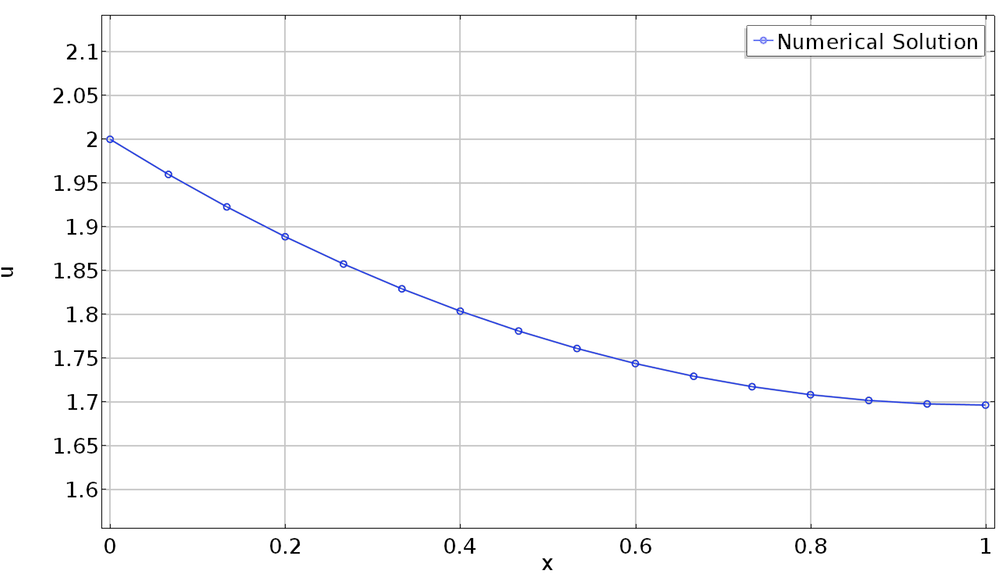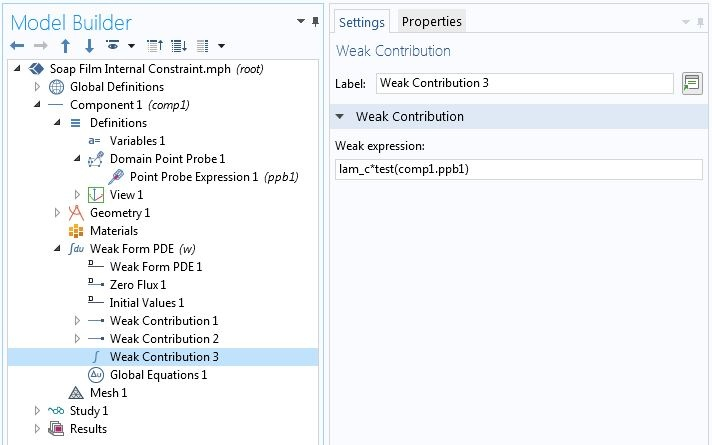# 在变分问题中指定边界条件和约束

2018年 9月 7日

### 等式约束理论简介

\mathcal{L}(x,\lambda) = f(\bf{x}) + \lambda g(\bf{x})

\textrm{求函数} u(x) \textrm {的最小值}
E[u(x)] = \int_a^b F(x,u,u^{\prime})dx,
\textrm{ 对于所有 x 满足} g(x,u,u^{\prime})=0 \textrm.

(1)

E[u(x),\lambda(x)] = \int_a^b [F(x,u,u’)+\lambda(x)g(x,u,u’)]dx.

\frac{d}{d\epsilon_1}E[u+\epsilon_1 \hat u,\lambda+\epsilon_2 \hat{\lambda}]\bigg|_{(\epsilon_1=0,\epsilon_2=0)} = 0, \quad
\frac{d}{d\epsilon_2}E[u+\epsilon_1 \hat u,\lambda+\epsilon_2 \hat{\lambda}]\bigg|_{(\epsilon_1=0,\epsilon_2=0)} = 0.

\int_a^b \left[\frac{\partial F}{\partial u}\hat{u} + \frac{\partial F}{\partial u’}\hat{u’} + \lambda(\frac{\partial g}{\partial u}\hat{u} + \frac{\partial g}{\partial u’}\hat{u’})\right]dx=0,
\int_a^b \left[\hat{\lambda}(x)g(x,u,u’)\right]dx=0.

\int_a^bg(x,u,u^{\prime})dx=G,

E[u(x),\lambda] = \int_a^b F(x,u,u^{\prime})dx+\lambda\left[\int_a^b g(x,u,u^{\prime})dx-G\right],

\int_a^b \left[\frac{\partial F}{\partial u}\hat{u} + \frac{\partial F} {\partial u’}\hat{u’}\right]dx + \lambda\int_a^b \left[\frac{\partial g}{\partial u}\hat{u} + \frac{\partial g}{\partial u’}
\hat{u’}\right]dx=0,
\hat{\lambda}\left[\int_a^b g(x,u,u’)dx-G\right]=0.

\int g(x,u,u^{\prime})\delta(x-x_o)dx=0.

(2)

\int_a^b \left[\frac{\partial F}{\partial u}\hat{u} + \frac{\partial F}{\partial u’}\hat{u’}
\right]dx + \lambda\left[\frac{\partial g}{\partial u}\hat{u} + \frac{\partial g} {\partial u’}
\hat{u’}\right]_{x=x_o}=0,

(3)

\hat{\lambda}g(x_o,u,u^{\prime})=0.

### 在 COMSOL Multiphysics® 中施加约束

\textrm{At } x=a, \frac{\partial g}{\partial u}=1, \frac{\partial g}{\partial u^{\prime}}=0,
\textrm{At } x=b, \frac{\partial g}{\partial u}=0, \frac{\partial g}{\partial u^{\prime}}=1• 对材料行为的约束
• 解的上限或下限
• 二维和三维问题中的边界条件

• 辅助因变量
• 弱贡献下的属性

• 边长
• 物体重量
• 表面平均位移（温度）

• 一维边界条件
• 约束在孤立的内部点上

• 辅助因变量
• 弱贡献下的属性

### 将约束添加到不在几何序列中的点### 查看“变分问题和约束”系列博客的更多博客文章

#### 评论 (1)

##### 留言##### Yanbin He
2021-02-21

This should be helpful for understanding the Dirichlet BCs. Can you show some examples about imposing continuity BCs?